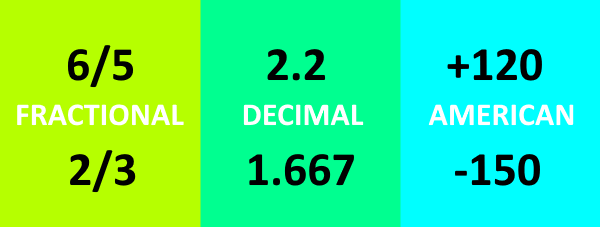# Sports Betting Odds Formats

There are three types of odds formats that are commonly used across the globe: American odds, decimal odds, and fractional odds. Many sportsbooks will give the bettor the option of which format they prefer. Is one format better than any other one? No, not really. However, understanding the differences between the three is an important tool to have in your sports betting repertoire.### Fractional Odds

Fractional odds are probably the easiest to understand for those who are new to sports betting. My first introduction into odds was on the horse track where fractional odds are still the king. In the United Kingdom, fractional odds are still the premier format as well.

The premise behind fractional odds is quite simple. To calculate the payout, one multiplies their bet by the odds value. So, if the price of a bet is 15/2 and the bet amount is \$100, then the payout would be \$850. This is equal to the \$100 wager plus the \$750 winnings. For another example, if the price of a bet is 1/4 and the bet amount is \$100, then the payout would be \$125. Again, here this represents the \$100 bet and \$25 in winnings.

### Decimal Odds

These odds are the most standard format of odds and are the primary format for most European countries. Decimal odds are the easiest to use once one gains an understanding of how they work. Simply, the decimal odds represent the multiplier for what the total payout (i.e. bet plus winnings) will be if the bet cashes. So, if the decimal odds on a bet are 2.99 and the bet amount is \$100, then the payout would be \$299. The breakdown for that payout calculation is \$100 for the bet and \$199 for the winnings.

There is no doubt that decimal odds seem to be complicated for those who are primarily used to American odds. However, I now prefer decimal odds over American odds as the calculations are easier and results more intuitive.

### American Odds

For most of the readers, American odds are the standard that they are used to. However, the format is arguably the most confusing of all three. If the odds amount is positive, then it represents the amount the bettor would win if \$100 were wagered. However, if the odds amount is negative, then the number represents how much must be wagered to win \$100.

For example, if the odds amount is +120 and the bettors wagers \$100, then the payout is \$220. The payout of \$220 comes from the \$100 bet plus the \$120 payout. Now, let’s say the odds amount is -200 and the bet amount is \$100. Here, we have to do some math, but we will find that the payout is \$150 (\$100 bet plus \$50 payout).

### Converting Odds Of Different Formats

There are a bunch of tools online that can do this for you. Our Odds Conversion Calculator does most of these conversions for you. In another example, the Action Network has a great odds conversion tool that will do all the heavy lifting for you. One more example can be found at AceOdds, where they have a great conversion tool and a few examples laid out side-by-side.